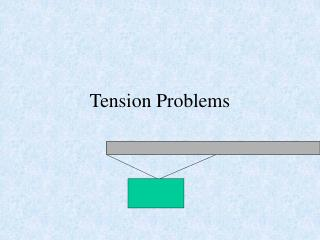DownloadDownload PresentationTension Problems

# Tension Problems

Télécharger la présentation## Tension Problems

- - - - - - - - - - - - - - - - - - - - - - - - - - - E N D - - - - - - - - - - - - - - - - - - - - - - - - - - -
##### Presentation Transcript

1. Tension Problems

2. What is Tension? • Tension is defined as a force transmitted along a rope, chain, or wire. • Tension will remain constant throughout the length of the rope. • Tension is treated as a force in force diagrams and calculations • Tension is measured in force units. (Newtons, dynes, or pounds (yuk!))

3. Mass is the amount of matter that an object is made up of, measured in g, kg, slugs The mass of an object remains constant at any location in the universe unless matter is added or removed Weight is the force of gravity acting on an object, measured in force units (N, dynes, or pounds) Weight depends on the acceleration due to gravity for the location of the object. Weight (w) = mg Weight or mass? What’s the difference?

4. Problems in Equilibrium • Since the object has no acceleration, the net force is equal to zero • The y-components of tension should be equal to the objects weight (Fy=0) • The forces in the x-direction should cancel each other (Fx=0)

5. Force Diagram(Free-Body Diagram) Ta Tb Tby=Tbsin2 Tay=Tasin1 1 2 Tax=Tacos1 Tbx=Tbcos2 W=mg

6. Applying Newton’s 2nd Law Since the sign is in equilibrium, the net force must be equal to zero. Fy = Ta sin θ1+Tb sin θ2-W = 0 Fy =Ta cos θ1 -Tb cos θ2 = 0 Ta Tb θ1 θ2 W=mg